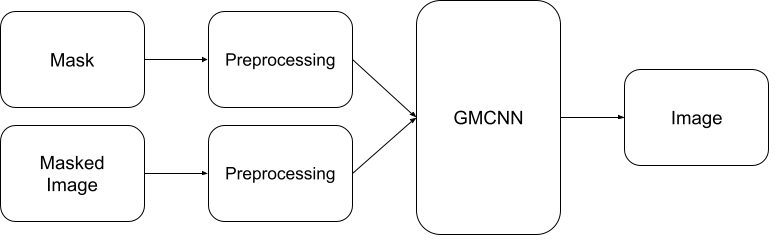# Image In-painting with OpenVINO™¶

This tutorial is also available as a Jupyter notebook that can be cloned directly from GitHub. See the installation guide for instructions to run this tutorial locally on Windows, Linux or macOS. To run without installing anything, click the “launch binder” button.

This notebook demonstrates how to use an image in-painting model with OpenVINO, using GMCNN model from Open Model Zoo. This model, given a tampered image, is able to create something very similar to the original image. The Following pipeline will be used in this notebook.```%pip install -q "openvino>=2023.1.0" "opencv-python" "matplotlib"
```
```[notice] A new release of pip is available: 23.2.1 -> 23.3.1
[notice] To update, run: pip install --upgrade pip
Note: you may need to restart the kernel to use updated packages.
```
```import sys
from pathlib import Path

import cv2
import matplotlib.pyplot as plt
import numpy as np
from zipfile import ZipFile
import openvino as ov

sys.path.append("../utils")
import notebook_utils as utils
```

Download `gmcnn-places2-tf`model (this step will be skipped if the model is already downloaded) and then unzip it. Downloaded model stored in TensorFlow frozen graph format. The steps how this frozen graph can be obtained from original model checkpoint can be found in this instruction

```# A directory where the model will be downloaded.
base_model_dir = "model"
# The name of the model from Open Model Zoo.
model_name = "gmcnn-places2-tf"

model_path = Path(f"{base_model_dir}/public/{model_name}/frozen_model.pb")
if not model_path.exists():
model_url = f"https://storage.openvinotoolkit.org/repositories/open_model_zoo/public/2022.1/gmcnn-places2-tf/{model_name}.zip"
else:

with ZipFile(f'{base_model_dir}/{model_name}' + '', "r") as zip_ref:
zip_ref.extractall(path=Path(base_model_dir, 'public', ))
```
```Already downloaded
```

## Convert Tensorflow model to OpenVINO IR format¶

The pre-trained model is in TensorFlow format. To use it with OpenVINO, convert it to OpenVINO IR format with model conversion API. For more information about model conversion, see this page. This step is also skipped if the model is already converted.

```model_dir = Path(base_model_dir, 'public', 'ir')
ir_path = Path(f"{model_dir}/frozen_model.xml")

# Run model conversion API to convert model to OpenVINO IR FP32 format, if the IR file does not exist.
if not ir_path.exists():
ov_model = ov.convert_model(model_path, input=[[1,512,680,3],[1,512,680,1]])
ov.save_model(ov_model, str(ir_path))
else:
```
```model/public/ir/frozen_model.xml already exists.
```

Now, load the OpenVINO IR model and perform as follows:

1. Initialize OpenVINO Runtime (Core).

2. Read the network from `*.bin` and `*.xml` files (weights and architecture)

3. Compile the model for the “CPU”.

4. Get input and output nodes.

Only a few lines of code are required to run the model:

```core = ov.Core()

# Read the model.xml and weights file
```
```import ipywidgets as widgets

device = widgets.Dropdown(
options=core.available_devices + ["AUTO"],
value='AUTO',
description='Device:',
disabled=False,
)

device
```
```Dropdown(description='Device:', index=2, options=('CPU', 'GPU', 'AUTO'), value='AUTO')
```
```# Load the model on to the device
compiled_model = core.compile_model(model=model, device_name=device.value)
# Store the input and output nodes
input_layer = compiled_model.input(0)
output_layer = compiled_model.output(0)
```

## Determine the input shapes of the model¶

Note that both input shapes are the same. However, the second input has 1 channel (monotone).

```N, H, W, C = input_layer.shape
```

Next, create a single channeled mask that will be laid on top of the original image.

```def create_mask(image_width, image_height, size_x=30, size_y=30, number=1):
"""
Create a square mask of defined size on a random location.

:param: image_width: width of the image
:param: image_height: height of the image
:param: size: size in pixels of one side
:returns:
"""

mask = np.zeros((image_height, image_width, 1), dtype=np.float32)
for _ in range(number):
start_x = np.random.randint(image_width - size_x)
start_y = np.random.randint(image_height - size_y)
pt1=(start_x, start_y),
pt2=(start_x + size_x, start_y + size_y),
color=(1, 1, 1),
thickness=cv2.FILLED)
```
```# Generate a square mask of size WxH with number of "holes".
# This mask will be laid over the input image as noise.
plt.figure(figsize=(16, 12))
```## Load and Resize the Image¶

This image will be altered by using the mask. You can process any image you like. Just change the URL below.

```img_path = Path("data/laptop.png")

if not img_path.exists():
url, filename="laptop.png", directory="data", show_progress=False, silent=True, timeout=30
)

# Resize the image to meet network expected input sizes.
resized_image = cv2.resize(src=image, dsize=(W, H), interpolation=cv2.INTER_AREA)
plt.figure(figsize=(16, 12))
plt.imshow(cv2.cvtColor(resized_image, cv2.COLOR_BGR2RGB));
```This multiplication of the image and the mask gives the result of the masked image layered on top of the original image. The `masked_image` will be the first input to the GMCNN model.

```# Generating a masked image.
plt.figure(figsize=(16, 12))
```## Preprocessing¶

The model expects the input dimensions to be `NHWC`.

• masked_image.shape = (512,680,3) —–> model expects = (1,512,680,3)

• resized_mask.shape = (512,680,1) —–> model expects = (1,512,680,1)

```masked_image = masked_image[None, ...]
```

## Inference¶

Do inference with the given masked image and the mask. Then, show the restored image.

```result = compiled_model([ov.Tensor(masked_image.astype(np.float32)), ov.Tensor(mask.astype(np.float32))])[output_layer]
result = result.squeeze().astype(np.uint8)
plt.figure(figsize=(16, 12))
plt.imshow(cv2.cvtColor(result, cv2.COLOR_BGR2RGB));
```## Save the Restored Image¶

```cv2.imwrite("data/laptop_restored.png", result);